Module 25 - Polar Functions Introduction | Lesson 1 | Lesson 2 | Lesson 3 | Self-Test Lesson 25.1: Polar Coordinates Polar coordinates are an alternative way of identifying points in a plane. They are useful in many applications and for certain types of regions and curves because functions given in polar coordinates can be simple to use. Polar coordinates will be explored in this lesson and you will learn how to convert points given in rectangular coordinates to polar coordinates and vice-versa. Defining Polar Coordinates When assigning rectangular coordinates (x, y) to a point P in the plane, x is the horizontal distance and y is the vertical distance from the origin O to the point P. If polar coordinates (r,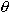) are used instead, r is the directed distance from the origin O to the point P andis the directed angle from the positive x-axis to ray OP. Recall that polar coordinates are not unique. In fact, there are infinitely many possible polar coordinates for any point in the plane.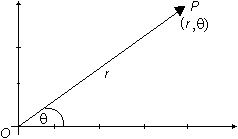Converting Rectangular Coordinates to Polar Coordinates The TI-89 has features that convert coordinates from rectangular to polar and vise-versa. Use the TI-89 to find a value of r for the point (3, 2). Set Angle mode to RADIAN Open the Math menu by pressing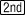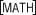Highlight "2:Angle"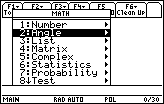Open the Angle submenu by pressing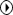Highlight "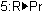("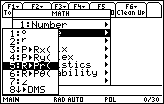This item returns the value of r in one of the possible polar coordinates for a point given in rectangular coordinates. Paste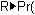to the Edit Line Enter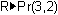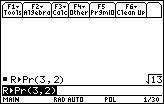The distance from the origin O to the point P is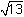, so the polar coordinate r is. Find a value offor this point. Open the Math Angle menu and highlight "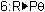("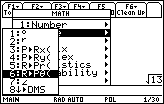Pressto paste this command to the Edit Line Complete the command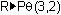and pressDisplay a decimal approximation by pressing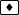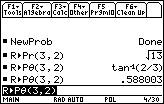The rectangular point (3, 2) is represented as (, arctan (2/3)), or approximately as (3.61, 0.588) in polar coordinates. Because the calculator is in Radian mode, the angle is given in radians. 25.1.1 If (r,) are polar coordinates for a point P, what are the other possible polar coordinates for P? Click here for the answer. Converting from Polar to Rectangular Coordinates 25.1.2 Use the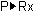and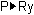features in the Math Angle menu to find the x- and y-coordinates for the polar point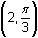. Click here for the answer. The rectangular coordinates for a point are unique. < Back | Next > ©Copyright 2007 All rights reserved. | Trademarks | Privacy Policy | Link Policy